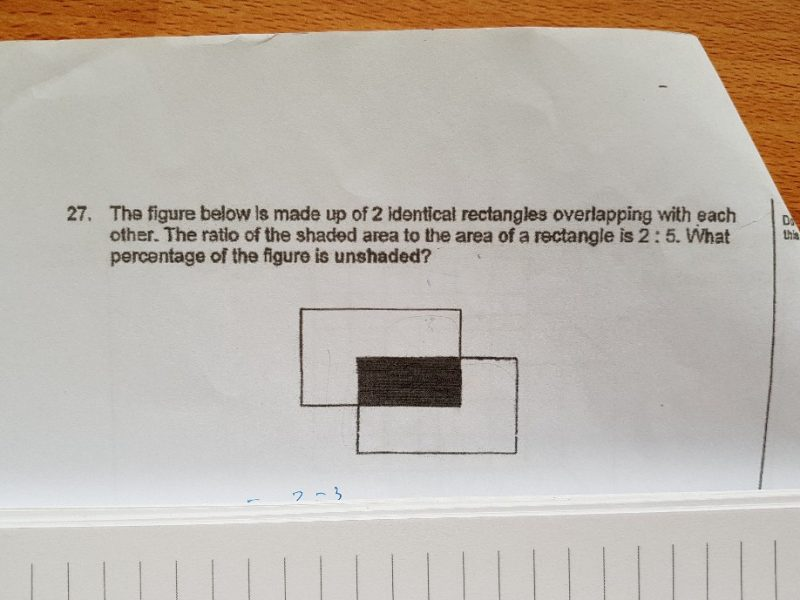# QuestionArea of a rectangle = 5 units

Therefore, unshaded part = 5 – 2 = 3 units.

For the figure, unshaded part = 3 + 3 = 6 units

Total area of figure = 6 + 2 = 8 units

Percentage that is shaded = 6 ÷ 8 × 100% = 75%